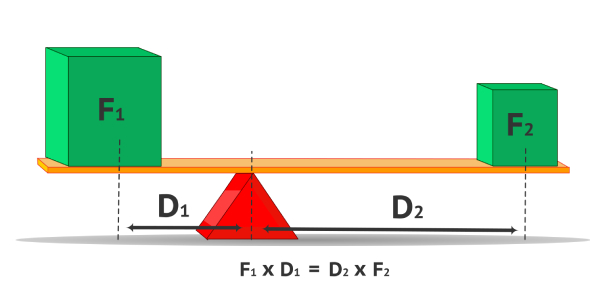# Quiz: Work, Power And Energy Questions

12 Questions | Attempts: 2856SettingsPractice quiz for chapter 7.

• 1.
A solar collector heats the water in the water tank of a home. Which energy transformation equation best describes this situation?
• A.

• B.

• C.

• D.

• E.

• 2.
The amount of work done when a 80.00-kg person jumps 1.000 m into the air in 0.5000 s is
• A.

292.0 J

• B.

40.00 J

• C.

784.00 J

• D.

1568 J

• E.

160.0 J

• 3.
The amount of work done to stop a bullet travelling through a tree trunk a distance of 50 cm with a force of 2.00 ´ 102 N is
• A.

–4.00 x 102 J

• B.

+4.00 x 102 J

• C.

+1.00 x 102 J

• D.

–1.00 x 102 J

• E.

+1.00 x 104 J

• 4.
A person lifts a pail of water of mass of 1.50 kg from the ground to a deck, 1.00 m above the ground. How much work was done by gravitational force on the pail of water?
• A.

–14.7 J

• B.

+1.50 J

• C.

–1.50 J

• D.

+0.153 J

• E.

+14.7 J

• 5.
In which case is negative work done?
• A.

The work done by Earth on a ball as the ball, which was initially at rest, falls 3.0 m down towards Earth.

• B.

A eastward force is applied to an eastward moving soccer ball that is already moving at a constant velocity to increase its speed in that direction.

• C.

A cart is moving at a constant velocity of 10 m/s [W] when a 0.5 N [downward] force is applied.

• D.

The work done by Earth on an arrow as it is fired 200 m straight up into the air.

• E.

Earth applies a force on the Moon as the Moon travels one completion rotation in orbit around Earth.

• 6.
In which case is positive work done?
• A.

The work done by air resistance on a ball as the ball, which was initially at rest, falls 3.0 m down towards Earth.

• B.

A eastward force is applied to an eastward moving soccer ball that is already moving at a constant velocity to increase its speed in that direction.

• C.

Earth applies a force on the Moon as the Moon travels one completion rotation in orbit around Earth.

• D.

A cart is moving at a constant velocity of 10 m/s [W] when a 0.5 N [downward] force is applied.

• E.

The work done by Earth on an arrow as it is fired 200 m straight up into the air.

• 7.
An elevator motor lifts a load of 2500 kg a height of 12.0 m in 3.00 seconds. The energy required by the motor to accomplish this task is 300 000 J. What is the efficiency of the elevator?
• A.

10.0%

• B.

98.0%

• C.

30.0%

• D.

102%

• E.

8.17%

• 8.
In the following figure, a 0.60-kg ball is about to fall to the step below.  The gravitational potential energy of the ball relative to the step is
• A.

8.8 x 101 J

• B.

2.9 x 101 J

• C.

6.0 x 100 J

• D.

3.0 x 100 J

• E.

5.9 x 101 J

• 9.
Jughead (m = 60 kg), Archie (m = 65 kg), and Reggie (m = 70 kg), all decide to run up a flight of stairs at with a height of 4.0 m at constant speed at good ol’ Riverdale High.  If all three achieve this task in a time of 3.5 seconds, who generates the most power?
• A.

• B.

Archie

• C.

Reggie

• D.

They all generate the same amount of power

• E.

Not enough information is provided to answer this question

• 10.
During a recent NBA basketball game, Jose Calderon, a guard with the Toronto Raptors, threw a basketball into the crowd in an unsportsmanlike manner.  Which one of the following statements about the basketball is FALSE?
• A.

As the basketball rises, its kinetic energy decreases

• B.

As the basketball rises, it is accelerated downward

• C.

As the basketball rises, its potential energy increases

• D.

When the basketball reaches the top of its flight, its kinetic energy is zero

• E.

When the basketball reaches the top of its flight, acceleration is still g

• 11.
Nicholas exerts a certain force to lift a dumbell 3.0 m straight above his head in 20 s. If he had completed the same task in 10 s, the energy he would need to use would be
• A.

Twice as great

• B.

The same

• C.

Four times as great

• D.

Half as great

• E.

Cannot be calculated using the given information

• 12.
If Elio pushes against an immobile wall with twice as much force as Jordan, which of the following is TRUE?
• A.

Elio is doing twice as much work as Jordan.

• B.

Elio generates twice as much power as Jordan.

• C.

Elio is doing four times as much work as Jordan.

• D.

No work is being done by Elio or Jordan.

• E.

They both generate power in equal amounts.

## Related TopicsBack to top
×

Wait!
Here's an interesting quiz for you.